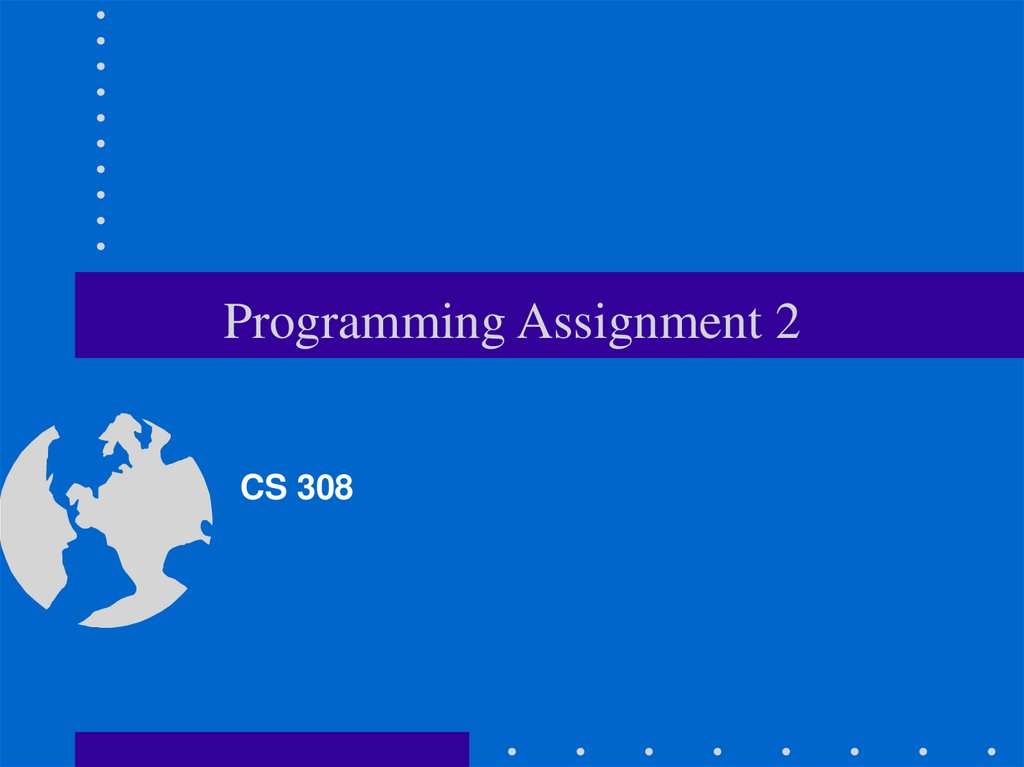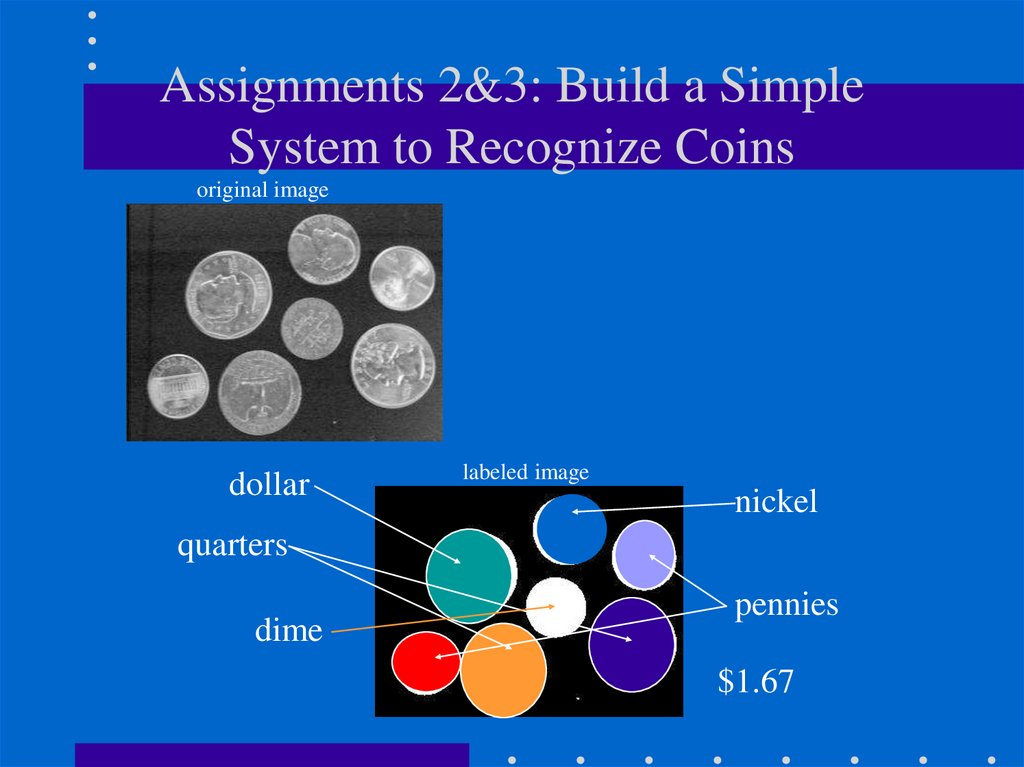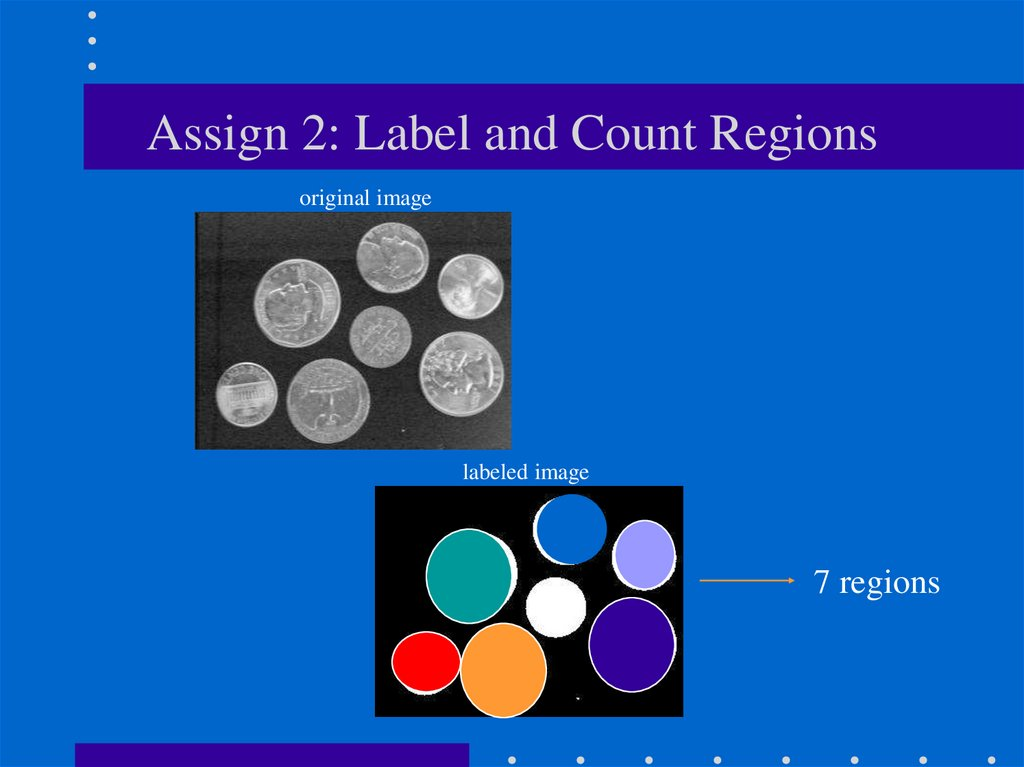# Programming Assignment

CS 308

## 2. Assignments 2&3: Build a Simple System to Recognize Coins

Assignments 2&3: Build a Simple
System to Recognize Coins
original image
dollar
labeled image
nickel
quarters
dime
pennies
\$1.67

original image
labeled image
7 regions

## 4. Project Objectives

• Improve your skills with manipulating stacks and queues.
• Improve your understanding of recursion.
• Illustrate how to convert a recursive algorithm to an
iterative one.
• Learn to document and describe your programs

## 5. Flowchart for labeling and counting regions

“Count/Label Regions”
(2) the steps given in the
diagram should be
executed when the user
selects this option.
(you can also have these steps

## 6. Thresholding

• Generates a binary (black/white) image of the input.
• Separates the regions corresponding to the coins from the background.
• Segmentation is useful for performing coin recognition:
– collect all the pixels belonging to the same region
– extract “features” useful for coin recognition

## 7. threshold(image, thresh)

• Implement it as a client function (only for grayscale images).
• Each pixel in the input image is compared against a threshold.
• Values greater than the threshold are set to 255, while values
less than the threshold are set to 0.
O(i, j )
255 if
0 if
I ( i , j ) T
I ( i , j ) T

original
thresholoded

## 9. Other Examples: Face segmentation

original
thresholded
candidate face regions

original
low threshold
good threshold
high threshold

## 11. displayHistogram(image)

• Implement it as a client function.
• The histogram is a bar graph of the pixel value frequencies (i.e.,
the number of times each value occurs in the image)

## 12. displayHistogram(image) -- cont’d

• Use an array of counters to store the pixel frequencies.
• Display the histogram as an intensity image.
– Draw a bar for every counter.
– Normalize counter values:
c
c
500
max_ c
500
0
0
255

## 13. Improving the results of thresholding

• In most cases, further processing is required to improve the
results of thresholding.
• For example, some of the regions in the thresholded image
might contain holes.

## 14. dilate(image) -- client function

Od (i, j )
255 if
I ( i , j ) if
at least one neighbor is 255
all 8 neighbors are 0

## 15. dilate(cont’d)

• Dilation “expands” the regions (i.e.,adds a layer of
boundary pixels)
original
thresholded
dilated

## 16. erode(image) -- client function

Oe (i, j )
255 if
I ( i , j ) if
all 8 neighbors are 255
at least one neighbor is 0

## 17. erode(image)

• Erosion “shrinks” the regions (i.e., removes a layer of
boundary pixels)
original
thresholded
eroded

## 18. Filling in the holes of regions

• Apply dilation to fill in the holes.
• Apply erosion to restore the size of the regions.
original
dilated
thresholded
eroded

## 19. Connected Components Algorithm

• Finds the connected components in an image and assigns a
unique label to all the points in the same component.

## 20. Connected Components Algorithm (cont’d)

1. Scan the thresholded image to find an unlabeled white
(255) pixel and assign it a new label L.
2. Recursively assign the label L to all of its 255 neighbors.
3. Stop if there are no more unlabeled 255 pixels.
4. Go to step 1
Print number of regions found.

## 22. int connectedComponents(inputImage, outputImage)

(client function)
int connectedComponents(inputImage, outputImage)
set outputImage --> 255 (white) // initialization
connComp=0;
for (i=0; i<N; i++)
for(j=0; j<M; j++)
if(inputImage[i][j] == 255 && outputImage[i][j]==255) {
++connComp;
label = connComp; // new label
findComponent( parameters ); // recursive function
// non-recursive functions
// findComponentDFS(inputImage, outputImage, i, j, label);
// findComponentBFS(inputImage, outputImage, i, j, label);
}
return connComp;

## 23. findComponent(parameters)

• Implement this as a recursive function.
• Think what the parameter list should be ...

• The main structure used used by BFS is the queue.
• BFS uses a queue to “remember” the neighbors of
pixel (i,j) that need to be labeled in future
iterations.
• The closest neighbors of (i,j) are labeled first.
• BFS will first label all pixels at distance 1 from
(i,j), then at distance 2, 3, etc.

## 25. findComponentBFS(inputImage, outputImage, i, j, label)

Queue.MakeEmpty();
Queue.Enqueue((i,j)); // initialize queue
while(!Queue.IsEmpty()) {
Queue.Dequeue((pi,pj));
outputImage[pi][pj] = label;
for each neighbor (ni,nj) of (pi,pj) // push neighbors
if(inputImage[ni][nj] == inputImage[pi][pj] && outputImage[ni][nj] == 255) {
outputImage[ni][nj] = -1; // mark this pixel
Queue.Enqueue((ni,nj));
}
}

1 1
1 1 1
1
P10
255
P1
dequeue
p3 p4
dequeue
P2 p10 p3 p4
dequeue
dequeue
p10 p3 p4
dequeue
p4 p5
dequeue
p5

## 27. Depth-First-Search (DFS)

• The main structure used used by DFS is the stack.
• DFS uses a stack to “remember” the neighbors of
pixel (i,j) that need to be labeled in future iterations.
• The most recently visited pixels are visited first (i.e.,
not the closest neighbors)
• DFS follows a path as deep as possible in the image.
• When a path ends, DFS backtracks to the most
recently visited pixel.

## 28. findComponentDFS(inputImage, outputImage, i, j, label)

Stack.MakeEmpty();
Stack.Push((i,j)); // initialize stack
while(!Stack.IsEmpty()) {
Stack.Pop((pi,pj));
outputImage[pi][pj] = label;
for each neighbor (ni,nj) of (pi,pj) // push neighbors
if(inputImage[ni][nj] == inputImage[pi][pj] && outputImage[ni][nj] == 255) {
outputImage[ni][nj] = -1; // mark this pixel
Stack.Push((ni,nj));
}
}

11
P10
255
1
1
1
1
pop
pop
P1
pop
pop
pop
pop
P4
P5
P6
P7
P3
P3
P3
P3
P3
P10
P10
P10
P10
P10
P2
P2
P2
P2
P2
etc.
1